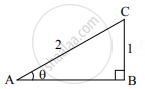# In the following figure, in ΔABC, BC = 1, AC = 2, ∠B = 90°. Find the value of sin θ. - Geometry

Sum

In the following figure, in ΔABC, BC = 1, AC = 2, ∠B = 90°. Find the value of sin θ.#### Solution

In ΔABC, m∠B = 90°,

thereforesintheta=

therefore sintheta=1/2Concept: Heights and Distances
Is there an error in this question or solution?